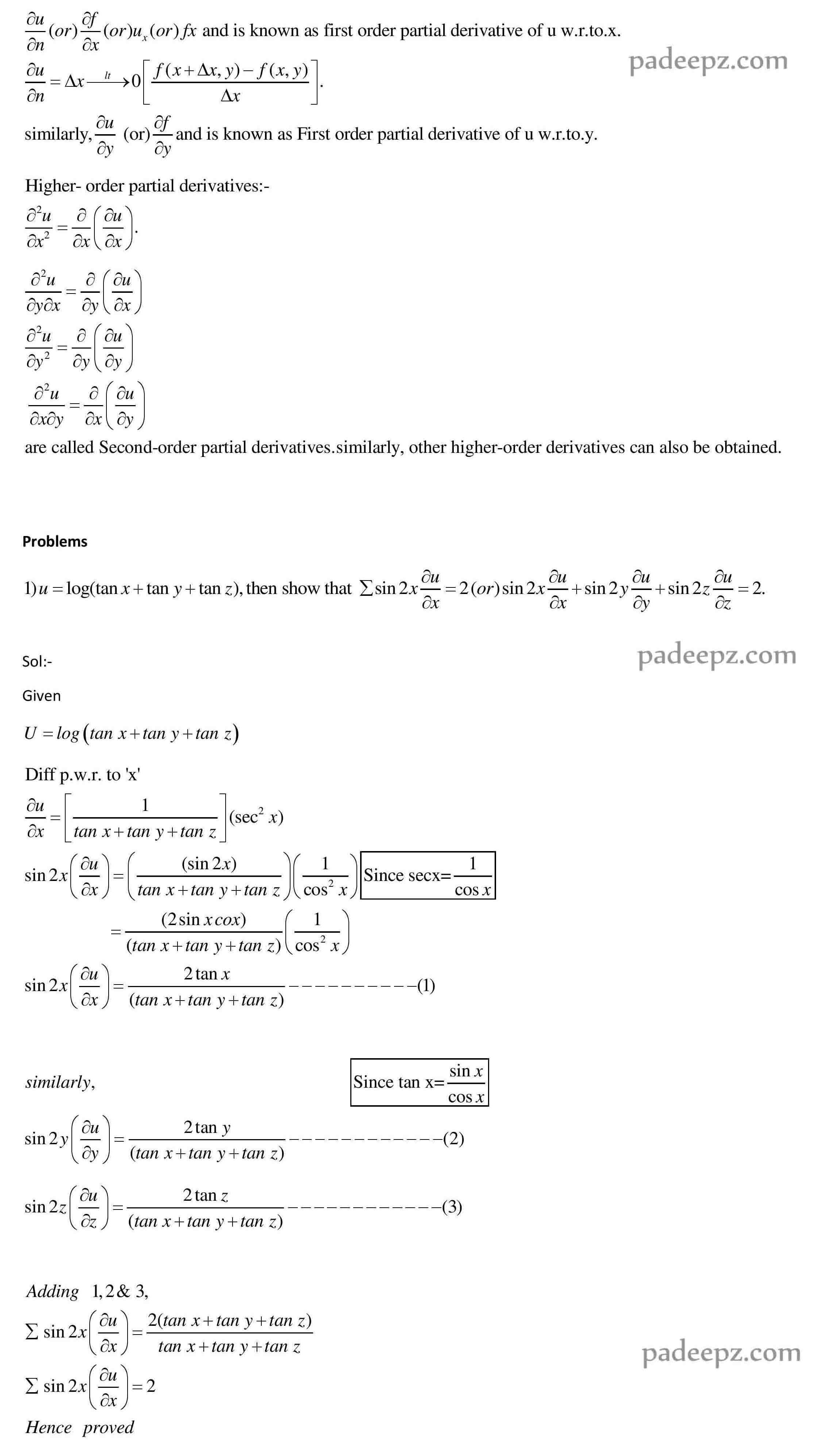# MA8151 Important questions Engineering Mathematics 1

## Sample MA8151 Important questions Engineering Mathematics 1:

PART-A MA8151 Important questions Engineering Mathematics 1

1. Find the domain and range of the function ?(?) = 1 ?2−?
2. Evaluate lim ?→2 ?2−5?+6 ?2+4
3. Show that lim ?→0|?| = 0
4. Where are of the function discontinues?         21 2 2 2 ) ( 2 ifx ifx x x x x f (MA8151 Important questions Engineering Mathematics 1)
5. Find ?? ?? if y = (2?3 + 3)( ?4 − 2?)
6. Find the equation of the tangent line to ? = 3? at (3,1)
7. Find ?′ if y = ???? 1−????
8. Find the critical point of ?(?) = ?3 + ?2 − ?

PART-B MA8151 Important questions Engineering Mathematics 1

1. Find the domain and range and sketch the graph of the function ?(?) = √? + 2
2. Evaluate lim ℎ→0 (3+ℎ)2−9 ℎ
3. Show that lim ?→0 ?2 ??? 1? = 0 using sandwich theorem.
4. Where the function ?(?) = ???? + ???−1? ?2−1 is continues?
5. Find ?? ?? if ? = sin (cos(????))
6. Find ?? ?? if sin(? + ?) = ?2???? (MA8151 Important questions Engineering Mathematics 1)
7. Find the absolute maximum and absolute minimum value of the function ?(?) = ?3 − 3?2 + 1 ?? 12 ≤ ? ≤ 4
8. Discuss the curve ?(?) = ?4 − 4?3 with respect to the local maximum and local minimum, concavity and the point of inflection.
9. If w =f ( ? − ?, ? − ?, ? − ? ) then show that . 0          zw yw xw
10. If z =f(x,y), Where , Prove that uv y v u x 2, 2 2    ) )( ( 42 2 2 2 2 2 2 2 2 2 yz xz v u vz uz        
11. If prove that          yx y x u 2 2 1 sin u yu y xu x tan    
12. Find the Jacobian of y1, y2, y3 with respect to x1, x2, x3 if , , . 1 3 2 1 xx x y  23 1 2 xx x y  3 1 2 3 xx x y  (MA8151 Important questions Engineering Mathematics 1)
13. Find the Taylor’s series expansion of ex cosy in the neighborhood of the point (1, π/4) upto the third degree terms
14. Expand ex log(1 + y) in powers of x and y upto terms of 3rd degree using Taylor’s expansion
15. Examine the function f(x, y) = x3 y2 (12 – x – y) for extreme values. (MA8151 Important questions Engineering Mathematics 1)
16. A rectangular box open at the top is to have a volume 32 c.c. Find the dimensions of the box requiring least material for its construction.

### Padeepz E-Learning Materials MA8151 Engineering Mathematics 1

Padeepz E-Learning Materials MA8151 Engineering Mathematics 1 we have provided the sample materials in this page. If you like the sample and want to buy the full subject the procedure is also provided in this page.

#### Partial derivatives

partial derivative Introduction:

A partial derivative of a function of several variables is the ordinary derivative with respect to one of the variables, when all the remaining variables are kept constant. Consider a function u=f(x,y). Here , u is the dependent variable and x & y are independent variables. The partial derivative of u=f(x,y) with respect to x is the ordinary derivative of u w.r.to x, keeping y constant. It is denoted bySubject name Engineering Mathematics 1 Subject Code MA8151 Semester 1 Regulation 2017 Regulation